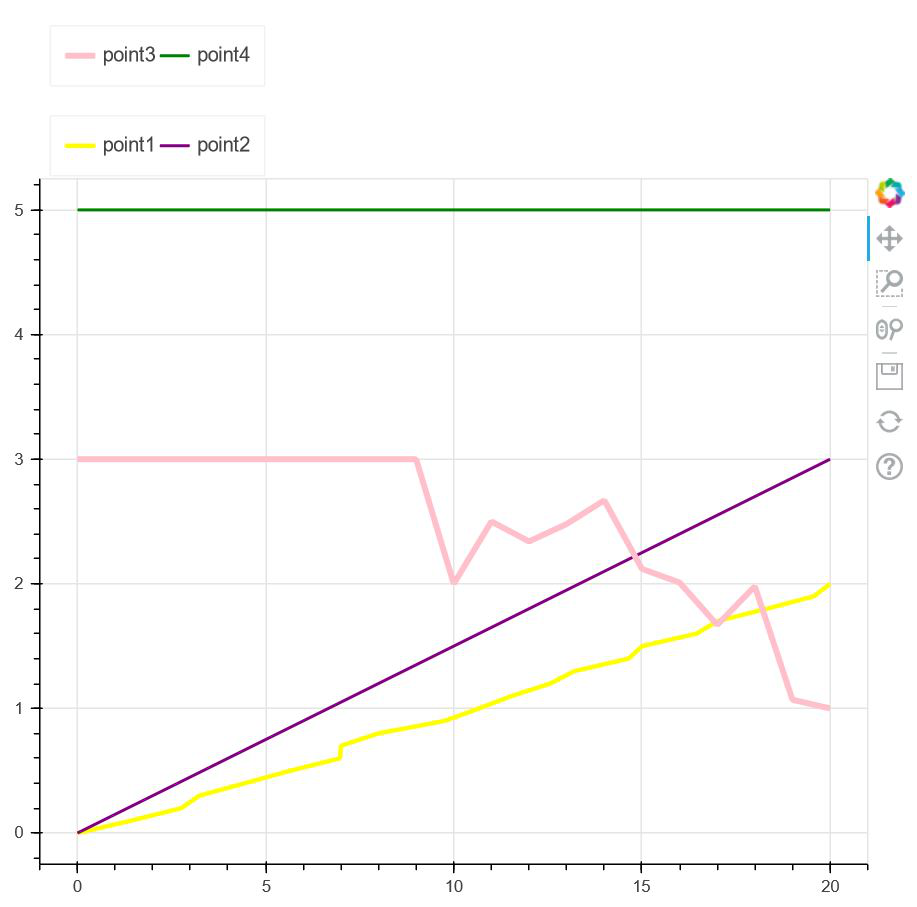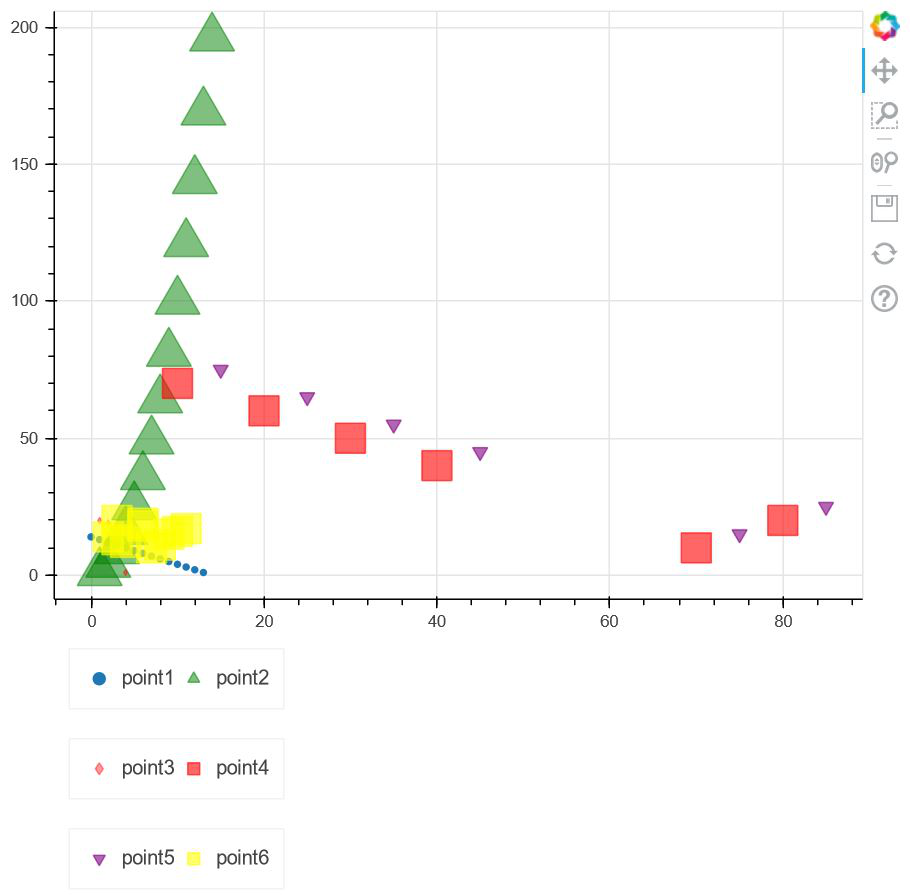Related Articles

# Create a two line legend in a Bokeh Plot

• Last Updated : 27 May, 2021

In this article, we will be learning about how to create a two line legend in the bokeh plot. Legends are one of the most essential parts of a bokeh plot. They help us to differentiate between different glyphs used in a plot. Other than that if we need to plot different lines of different colors in the same plot, we can differentiate them using legend. Also, we can change various properties of the things mentioned in the legend.

But before we dive deep into the topic, we should either have google colab or should be using a local device code editor. If we are working in google colab, then we can straight away go to the below implementation. But if we are using a local device, then we should ensure that we have bokeh preinstalled in our device, else we can install it too. Open a command prompt and write

`pip install bokeh`

Now, everything is set. The installation is essential in our local device else the functionalities won’t work. Let’s move to the above implementation.

Example 1:

In the first example, we are looking at some lines which are plotted using a wide range of points in an empty canvas. Using legend in bokeh we are differentiating the lines. The main thing to notice here is, we are having a two-line legend. So without further wait, let’s go to the code and see how it works.

Code:

## Python3

 `# importing show from``# bokeh.io to show the plot``from` `bokeh.io ``import` `show` `# importing legend from bokeh.models``from` `bokeh.models ``import` `Legend` `# importing figure from bokeh.plotting``from` `bokeh.plotting ``import` `figure` `# importing numpy package from python``import` `numpy as np` `# Creating an empty figure of plot height=600``# and plot width=600``fig ``=` `figure(plot_height``=``600``, plot_width``=``600``)` `# Creating point1 which is a yellow color line with``# line width as 3 for a set of points``point1 ``=` `fig.line(x``=``[``0``, ``1.43``, ``2.76``, ``3.24``, ``4.45``, ``5.65``,``                     ``6.98``, ``7``, ``8``, ``9.76``, ``10.67``, ``11.54``, ``12.567``,``                     ``13.21``, ``14.65``, ``15``, ``16.45``, ``17``, ``18.32``, ``19.57``, ``20``],``                  ``y``=``np.linspace(``0``, ``2``, ``21``),``                  ``line_width``=``3``, color``=``"yellow"``)` `# Creating point2 which is a purple color line with``# line width as 2 for a set of points``point2 ``=` `fig.line(x``=``np.linspace(``0``, ``20``, ``20``), y``=``np.linspace(``0``, ``3``, ``20``),``                  ``line_width``=``2``, color``=``"purple"``)` `# Creating point3 which is a pink color line with``# line width as 4 for a set of points``point3 ``=` `fig.line(x``=``[``0``, ``1``, ``2``, ``3``, ``4``, ``5``, ``6``, ``7``, ``8``, ``9``, ``10``, ``11``,``                     ``12``, ``13``, ``14``, ``15``, ``16``, ``17``, ``18``, ``19``, ``20``],``                  ``y``=``[``3``, ``3``, ``3``, ``3``, ``3``, ``3``, ``3``, ``3``, ``3``, ``3``, ``2``, ``2.5``, ``2.34``,``                     ``2.48``, ``2.67``, ``2.12``, ``2.01``, ``1.67``, ``1.98``, ``1.07``, ``1``],``                  ``line_width``=``4``, color``=``"pink"``)` `# Creating point4 which is a green color line with``# line width as 2 for a set of points``point4 ``=` `fig.line(x``=``[``0``, ``20``], y``=``5``,``                  ``line_width``=``2``,``                  ``color``=``"green"``)` `# Using legend we are placing two point descriptions``# beside each other horizontally``legend1 ``=` `Legend(items``=``[(``"point1"``, [point1]),``                        ``(``"point2"``, [point2])],``                 ``location``=``(``7``, ``2``), orientation``=``"horizontal"``)` `# Using legend we are placing th other two point``# descriptions beside each other horizontally``legend2 ``=` `Legend(items``=``[(``"point3"``, [point3]),``                        ``(``"point4"``, [point4])],``                 ``location``=``(``7``, ``2``), orientation``=``"horizontal"``)` `# placing legend1 at the top``fig.add_layout(legend1, ``'above'``)` `# placing legend2 at the top``fig.add_layout(legend2, ``'above'``)` `# showing the figure``show(fig)`

Output:Explanation:

• In the above example, we are importing all the necessary packages at first such as show, figure, Legend, and numpy from different modules of the bokeh library that python provides us.
• After importing the packages, we are creating an empty figure of plot width and height as 600.
• After that, we are creating four different lines with a different set of points along with different line colors and line width. In order to identify them, a legend is used.
• Now to create a two-line legend, we are using two variables legend1 and legend2 where we are storing two points in each of the variables where we are providing the orientation as horizontal. As a result, two will be shown in each row.
• Using ‘add_layout‘ we are determining the position of the legend as above i.e it will be shown at the top of the graphical representation.
• Finally, we are showing the plot using a show from bokeh.io.

Example 2:

In the next example, we will be exploring the concept with some glyphs provided by bokeh. Bokeh provides us with various types of glyphs, so we will be implementing the same concept used in the earlier example.

Code:

## Python3

 `# importing show from``# bokeh.io to show the plot``from` `bokeh.io ``import` `show` `# importing legend from bokeh.models``from` `bokeh.models ``import` `Legend` `# importing figure from bokeh.plotting``from` `bokeh.plotting ``import` `figure` `# importing numpy package from python``import` `numpy as np` `x1 ``=` `[``7``, ``8``, ``4``, ``3``, ``2``, ``9``, ``10``, ``11``, ``6``, ``6``, ``3``]``y1 ``=` `[``10``, ``11``, ``12``, ``13``, ``14``, ``15``, ``16``, ``17``, ``18``, ``19``, ``20``]` `# Creating an empty figure of plot height=600``# and plot width=600``fig ``=` `figure(plot_height``=``600``, plot_width``=``600``)` `# Creating point1 in the form of circular glyphs``# with a set of points``point1 ``=` `fig.circle(x``=``np.arange(``14``),``                    ``y``=``[``14``, ``13``, ``12``, ``11``, ``10``, ``9``, ``8``, ``7``,``                       ``6``, ``5``, ``4``, ``3``, ``2``, ``1``])` `# Creating point2 in the form of triangular glyphs``# with a set of points``point2 ``=` `fig.triangle(x``=``[``1``, ``2``, ``3``, ``4``, ``5``, ``6``, ``7``, ``8``, ``9``, ``10``, ``11``,``                         ``12``, ``13``, ``14``],``                      ` `                      ``y``=``[``1``, ``4``, ``9``, ``16``, ``25``, ``36``, ``49``, ``64``, ``81``, ``100``,``                         ``121``, ``144``, ``169``, ``196``], size``=``30``,``                      ` `                      ``color``=``"green"``, alpha``=``0.5``)` `# Creating point3 in the form of diamond shaped glyphs``# with a set of points``point3 ``=` `fig.diamond(x``=``[``1``, ``2``, ``4``],``                     ``y``=``[``20``, ``19``, ``1``],``                     ``color``=``"red"``,``                     ``alpha``=``0.4``)` `# Creating point4 in the form of square shaped glyphs``# with a set of points``point4 ``=` `fig.square(x``=``[``70``, ``80``, ``40``, ``30``, ``20``, ``10``],``                    ``y``=``[``10``, ``20``, ``40``, ``50``, ``60``, ``70``],``                    ``color``=``"red"``, size``=``20``,``                    ``alpha``=``0.6``)` `# Creating point5 in the form of inverted triangular glyphs``# with a set of points``point5 ``=` `fig.inverted_triangle(x``=``[``75``, ``85``, ``45``, ``35``, ``25``, ``15``],``                               ``y``=``[``15``, ``25``, ``45``, ``55``, ``65``, ``75``],``                               ``color``=``"purple"``, size``=``10``, alpha``=``0.6``)` `# Creating point6 in the form of square shaped glyphs``# with a set of points``point6 ``=` `fig.square(x1, y1, color``=``"yellow"``,``                    ``size``=``20``, alpha``=``0.6``)` `# Using legend we are placing two point descriptions``# beside each other``# horizontally``legend1 ``=` `Legend(items``=``[(``"point1"``, [point1]),``                        ``(``"point2"``, [point2])],``                 ``location``=``(``10``, ``10``), orientation``=``"horizontal"``)` `# Using legend we are placing th other two point``# descriptions beside each other horizontally``legend2 ``=` `Legend(items``=``[(``"point3"``, [point3]), (``"point4"``, [point4])],``                 ``location``=``(``10``, ``10``), orientation``=``"horizontal"``)` `# Using legend we are placing th other two point``# descriptions beside each other horizontally``legend3 ``=` `Legend(items``=``[(``"point5"``, [point5]), (``"point6"``, [point6])],``                 ``location``=``(``10``, ``10``), orientation``=``"horizontal"``)` `# placing legend1 at the bottom``fig.add_layout(legend1, ``'below'``)` `# placing legend2 at the bottom``fig.add_layout(legend2, ``'below'``)` `# placing legend3 at the bottom``fig.add_layout(legend3, ``'below'``)` `# showing the figure``show(fig)`

Output:Explanation:

• After importing the required packages, we are taking two variables x1 and y1 with a set of points that are to be plotted later.
• After that, we are setting the plot width and plot height as 600. Now, bokeh provides us with a variety of glyphs that can be used to differentiate different plots in a graph. So, we took some of the glyphs to point 6 such graphs in our plot with a wide range of points.
• After pointing them, we used the legend to identify each of the glyphs and we kept two points beside each other along with their orientation as ‘horizontal‘.
• Now using ‘add_layout‘ option, we can determine the position of the legend box. In this case, we are placing it at the bottom.
• Finally, we are showing the figure with the help of ‘show‘ from ‘bokeh.io‘.

Attention geek! Strengthen your foundations with the Python Programming Foundation Course and learn the basics.

To begin with, your interview preparations Enhance your Data Structures concepts with the Python DS Course. And to begin with your Machine Learning Journey, join the Machine Learning – Basic Level Course

My Personal Notes arrow_drop_up